# Circle Theorems

?
• Created by: DJSalt
• Created on: 02-03-17 16:08

## Circle theorem 1

Angles at the centre are double the angle at the circumference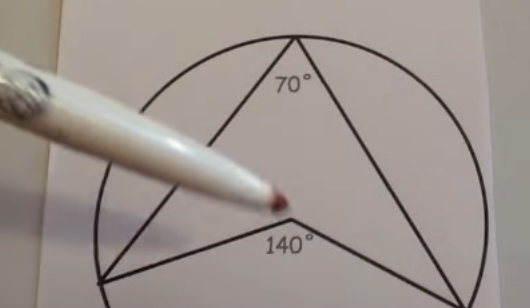1 of 8

## Circle theorem 2

Angles in a semi-circle are 90 degrees.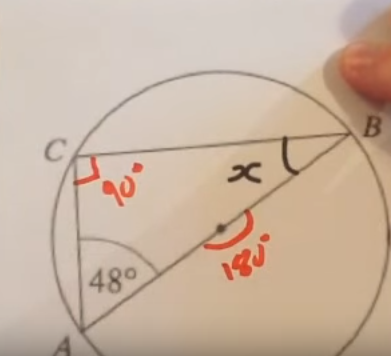2 of 8

## Circle theorem 3

Angles in the same segment are equal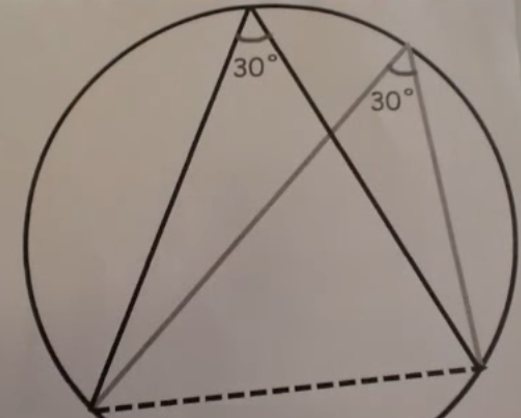3 of 8

## Circle theorem 4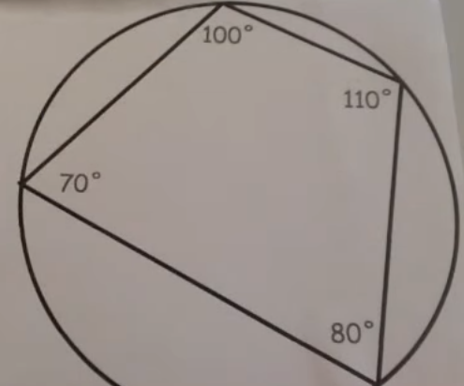4 of 8

## Circle theorem 5

Lengths of tangents are equal if from the same point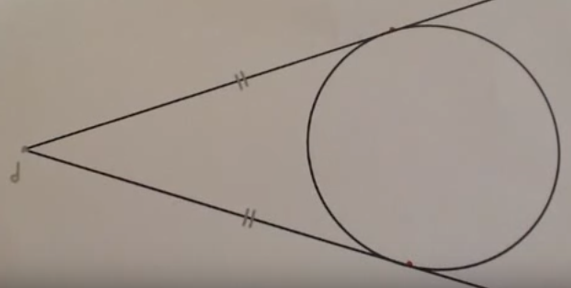5 of 8

## Circle theorem 6

The angle between the radius and the tangent is 90 degrees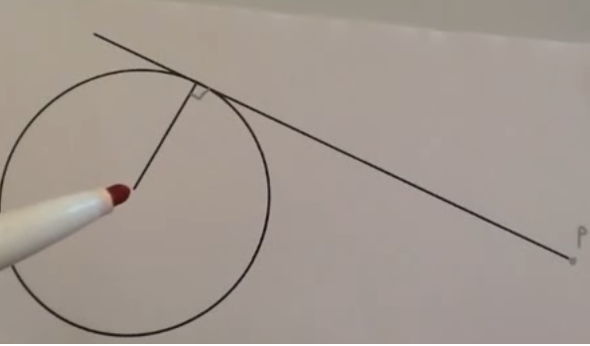6 of 8

## Circle theorem 7

The angle between the tangent and chord is equal to the angle in the alternate segment. This is called alternate segment theorem.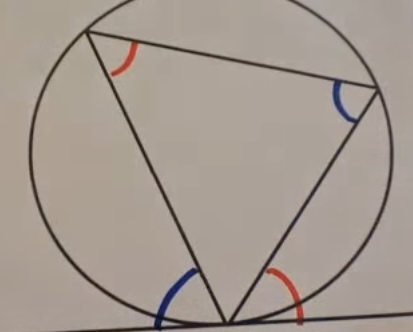7 of 8

## Circle theorem 8

Radius may bisect the chord which can produce a right angle.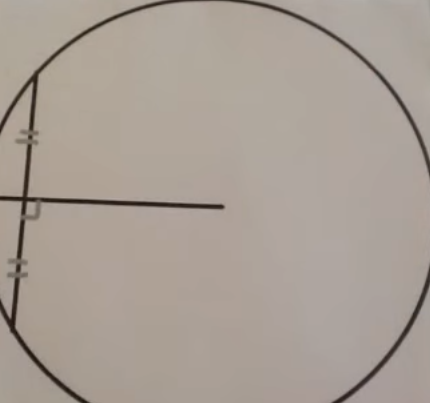8 of 8